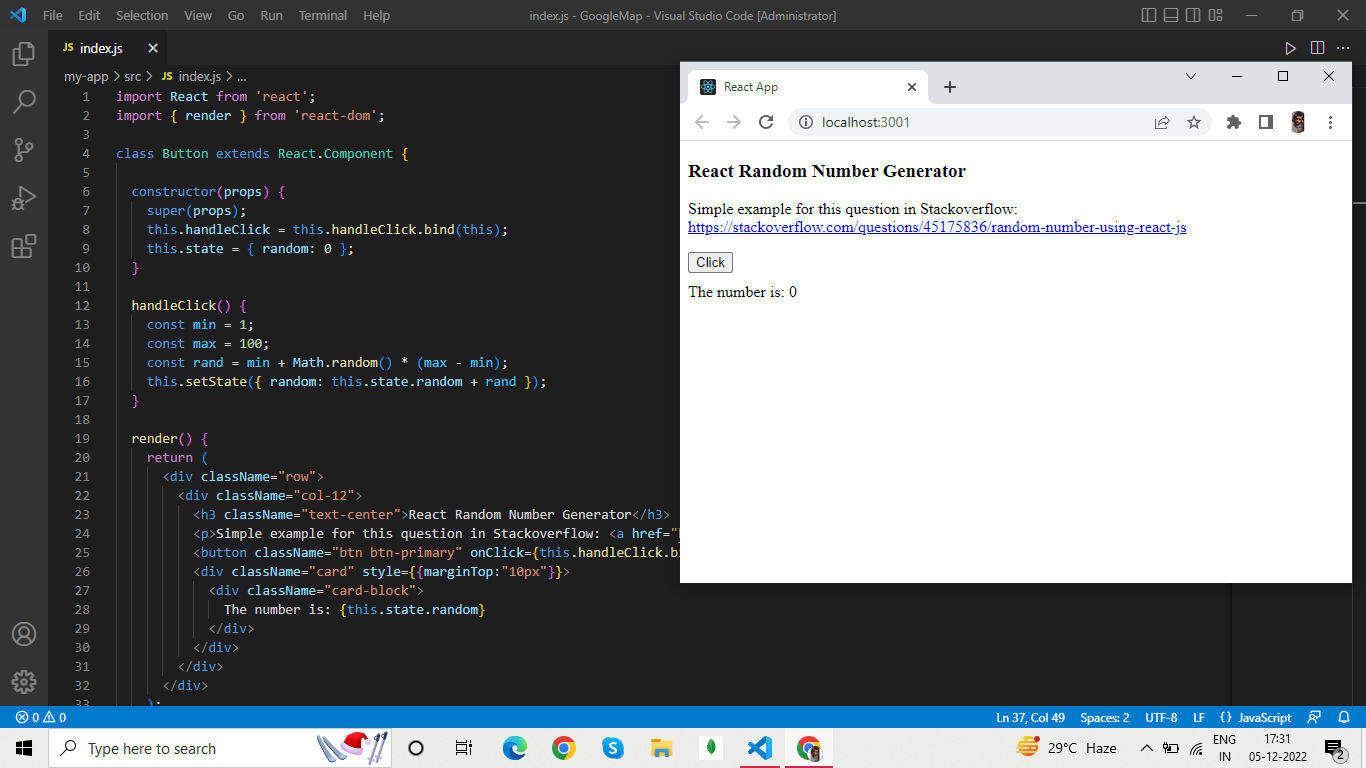# How to Generate a Random Number using ReactJSby Abdul Rawoof A RUpdated: Feb 1, 2023Solution Kit

A random number generator is a process or device that generates a sequence of random numbers. These numbers are often generated by a computer algorithm. Statistical analysis or simulations, including computer games or the simulating of physical processes, frequently employ the numbers produced by a random number generator. A wide range of applications can benefit from random number generators since they can offer a supply of unexpected data that can be used to test algorithms or models.

We can use JavaScript's built-in Math object to do further operations on the generated random number. To construct a random number generator in React, use the Math.random() function.

• Math.random(): This built-in function, produces a random integer between 0 and 1. The resultant number, for instance, 0.123456, is a floating-point value with a decimal. You may combine the Math.random() method with additional JavaScript functions to produce random integers inside a certain range.
• Math.floor(): This method is frequently used to round integers down to the closest integer.

To know more about Random Number generator in React, refer to the code given below.Fig : Preview of the output that you will get on running this code from your IDE.

### Code

In this solution we are using React library.

### Instructions

Follow the steps carefully to get the output easily.

1. Install the Node.js and React on your IDE(preferable Visual Studio Code).
2. Create React Application using npx create-react-app foldername.
3. cd foldername.
4. Open the folder in IDE.
5. Copy the code using "copy" button above and paste it in index.js file(remove the earlier code from index.js).
6. Open the terminal from IDE.
7. npm start to run the file.

You can also refer this url 'DEMO' for getting the above output.

I hope you found this useful. I have added the link to dependent libraries, version information in the following sections.

I found this code snippet by searching for 'how to create random number generator using reactjs' in kandi. You can try any such use case!

### Environment Tested

I tested this solution in the following versions. Be mindful of changes when working with other versions.

1. The solution is created in Visual Studio Code 1.73.1.
2. The solution is tested on node v18.12.1 and npm v8.19.2.
3. React version-18.2.0.

Using this solution, we are able to create random number generator using reactjs with simple steps. This process also facilities an easy way to use, hassle-free method to create a hands-on working version of code which would help us to create random number generator using reactjs.

### Dependent Library

JavaScript209050Version:v18.2.0The library for web and native user interfaces

Support
Quality
Security
Reuse

JavaScript209050Version:v18.2.0License: Permissive (MIT)

The library for web and native user interfaces
Support
Quality
Security